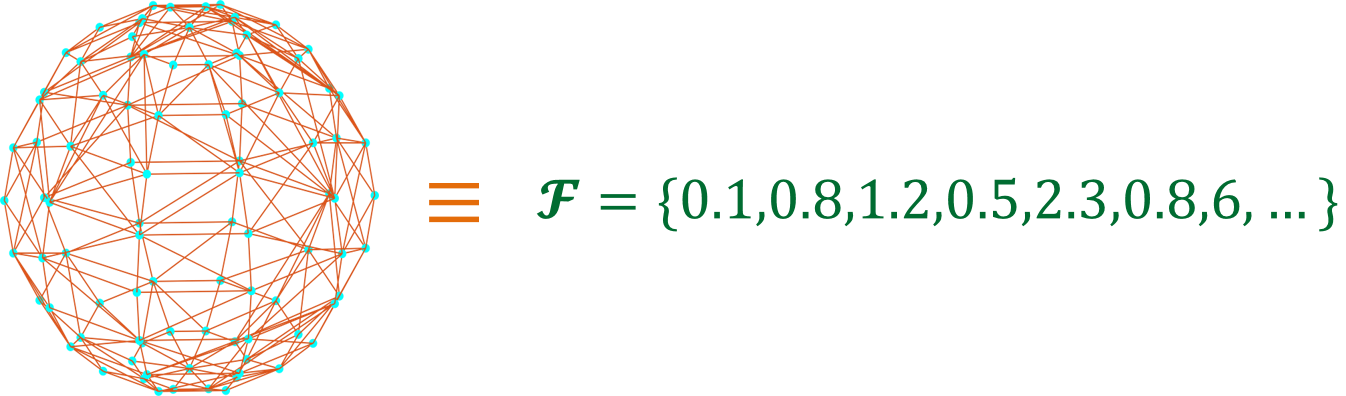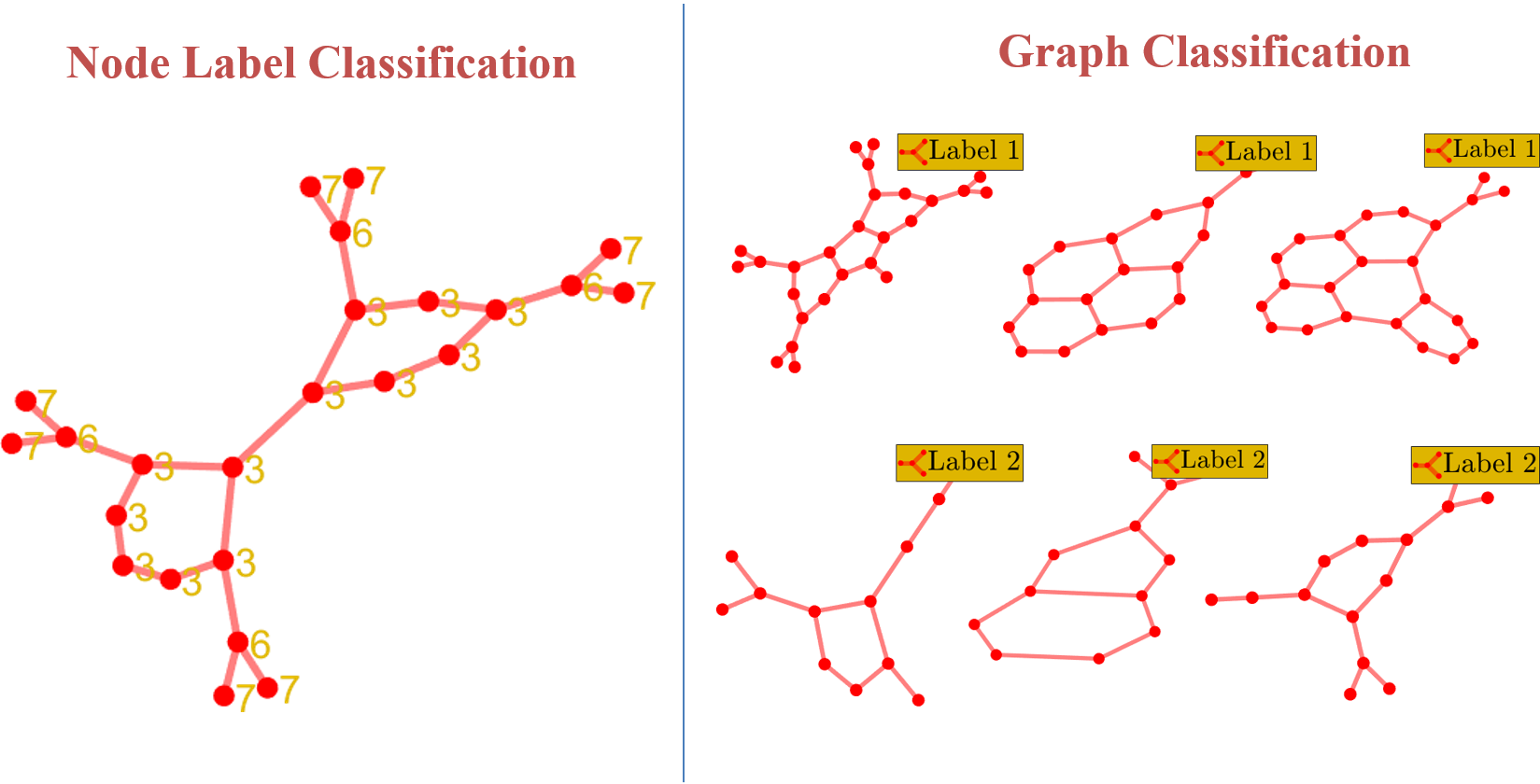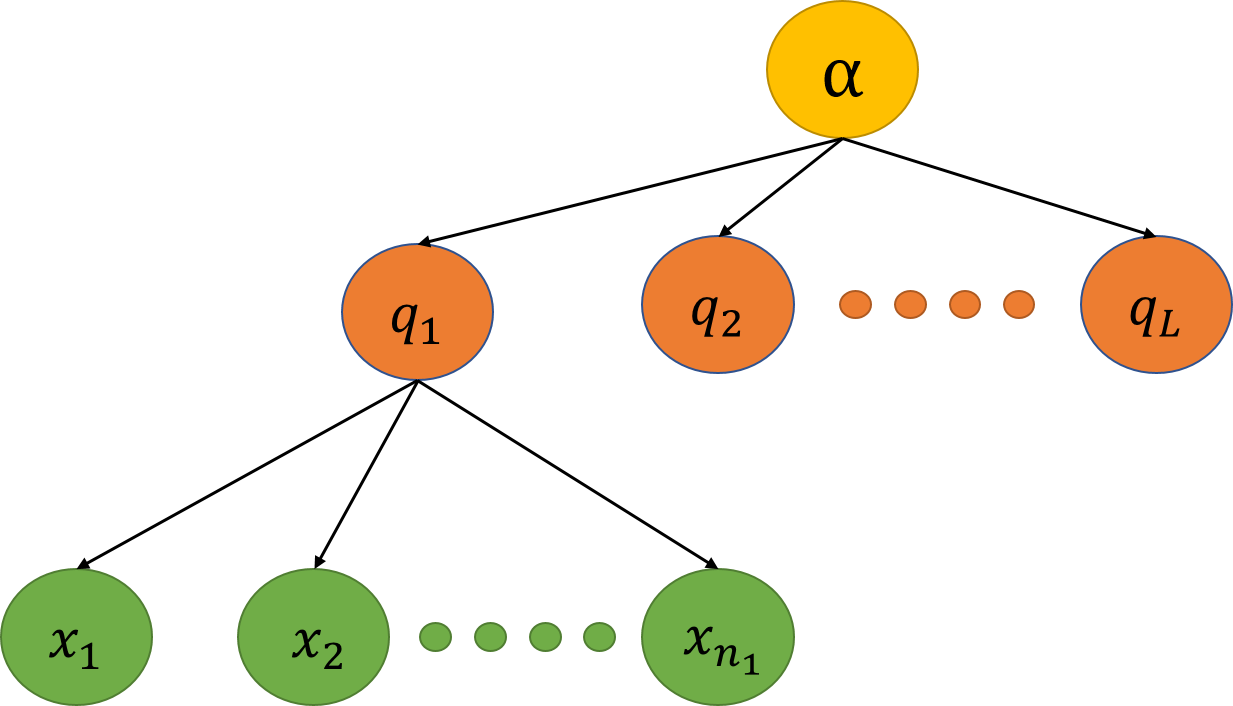# Blog: Machine Learning Equations by Saurabh Verma

## Blog: Hunt For The Unique, Stable, Sparse And Fast Feature Learning On Graphs (NIPS 2017).

• TutorialsHow can we convert a graph into a Feature Vector?

Graph is a fundamental but complicated structure to work with from machine learning point of view. Think about it, a machine learning model usually require inputs in some form of mathematical objects like vectors, matrices, real number sequences and then produces the desired outputs. So, the question is how we can convert a graph into a mathematical object that is suitable for performing machine learning tasks such as classification or regression on graphs.

For past one year, I have been working on developing machine learning models for graph(s) in order to solve two specific problems:

1) Graph Classification: Given a collection of graphs $\{G_i\}_{i=1}^{M}$ and associated graph labels $\{y_i\}_{i=1}^{M}$ where $y_i\in \mathbf{R^{c}}$ ($c$ is the number of classes), we need to come up with a learning model or function $F:G \rightarrow Y$ that can classify or predict the label associated with a graph.

2) Node Classification: Given a graph $G=(V,E,W)$, where $V$ is vertex set, $E$ is edge set & $W$ is adjacency matrix and labels $\{y_i\}_{i=1}^{M}$ where $y_i\in \mathbf{R^{c}}$ associated with each node, our task is to classify or predict the labels on each node. That is, come up a learning function $F:V\rightarrow Y$.## Blog: Stability of Learning Algorithms Part 3.

• Tutorials

Extending to Ranking Algorithms

## Blog: Stability of Learning Algorithms Part 2.

• Tutorials

Extending to Randomized Algorithms

Let $R$ be a set of random parameters.

Let’s assume that our algorithm $A_{(S,R)}$ has uniform stability $(\beta,\rho)$, if it satisfies,

## Blog: Stability of Learning Algorithms Part 1.

• Tutorials

This is walk-through on how to derive generalization bounds based on the stability of algorithms. Understanding the proof helps to extend or modify the results according to your needs. For instance, I had a loss function which was unbounded, and since to apply generalization results your loss function must be bounded, I was stuck. So, this my effort to state the proof as clear as possible along with the assumptions needed.

## Blog: Dirichlet Distributions.

• Tutorials

Dirichlet Distribution is a distribution over distributions. More specifically, it is a distribution over pmfs (probability mass functions). You can imagine, as if there is a bag of $L$ dices, and each dice has a corresponding pmf (related to six possible outcomes). Now picking a dice is like sampling a particular pmf from a distribution. The probability of picking a dice, which results in a pmf, comes from the Dirichlet distribution $Dir(\boldsymbol\alpha)$.

Let $\mathbf{q}=[q_1,q_2,...,q_k]$ be a pmf (or a point in simplex $\in$ $\mathbf{R}^{k}$), where $\sum\limits_{j=1}^{k}q_j=1$ and $\mathbf{q} \sim Dir(\boldsymbol\alpha)$. Here $\boldsymbol\alpha$ is dirichlet parameter $\boldsymbol\alpha=[\alpha_1,\alpha_2,...,\alpha_k]$ and $\alpha_0=\sum\limits_{j=1}^{k}\alpha_j$. Then, the probability density of $\mathbf{q}$ is given by:

Graphical Model:Suppose $\{x_i\}$ is a set of samples drawn from $i^{th}$ pmf where $i\in[1,L]$. For eg. $\{x_i\}$ could be a sequence of outputs of a dice $\{1,2,1,3,4,3,6,..\}$. Let $\mathbf{q}_1,\mathbf{q}_2,...,\mathbf{q}_L$ are $L$ pmfs. Then:

Let $n_{ij}$ be the number of outcomes in $\{x_i\}$ sequence of samples that is equal to $j^{th}$ event where $j\in[1,k]$, and let $n_i = \sum\limits_{j=1}^{k} n_{ij}$.

Therefore,

## Blog: Bayesian Sets.

• Tutorials

Bayesian Sets Graphical Model:Bayesian sets are simple graphical models used to expand a set. For instance, suppose you are given a set of few words (or items) $S=\{cat, dog, lion, ...\}$ which we refer as “seeds” and we wish to expand this set to include all similar words from the given text corpus. Then, we can employ Bayesian sets which rank each item based on its importance of belonging to seed set.

Let $D$ be a data set of items, and $\mathbf{x} \in D$ be an item from this set. Assume the user provides a query set $D_c$ which is a small subset of $D$, bayesian sets rank each item by $score(\mathbf{x})$. This probability ratio can be interpreted as the ratio of the joint probability of observing $x$ and $D_c$ to the probability of independently observing $x$ and $D_c$.

Assume that the parameterized model is $p(\mathbf{x} \vert \theta)$ where $\theta$ are the parameters as shown in figure above. Here, we assume that $\mathbf{x}$ is represented by a binary feature vector and $\theta_j$ is the weight associated with feature $j$. Then,

For each $\mathbf{x}_i$ (Note: $\mathbf{x}$ vector $j^{th}$ component is $x_{.j}$ and bold letters are vectors):

The conjugate prior for the parameters of a Bernoulli distribution is the Beta distribution:

## Blog: Why Regularized Auto-Encoders learn Sparse Representation?

• PaperList

This paper has some great insights to offer in design of autoencoders. As title suggests, it addresses “whether regularized helps in learning sparse representation of the data theoretically?”

Here is the setup: we have an input $\mathbf{x} \in \mathbf{R}^{d}$ which is mapped to latent space $\mathbf{h=s(Wx+b)}$ via autoencoder where $\mathbf{s}$ is encoder activation function, $\mathbf{W} \in \mathbf{R}^{m\times n}$ is the weight matrix, and $\mathbf{b} \in \mathbf{R^m}$ is the encoder bias and $\mathbf{h} \in \mathbf{R^m}$ is hidden representation or outputs of hidden units.

For analysis, paper assumes that decoder is linear i.e. $\mathbf{W^{T}h}$ decodes back the encoded hidden representation and loss is squared loss function. Therefore, for learning $\mathbf{W,b}$ parameters of autoencoder; objective function is:

Now we are interested in sparsity of $\mathbf{h}$, hidden representation.

Besides above, paper makes two more assumptions.

Assumption 1: Data is drawn from a distribution $\mathbf{x} \sim X$ for which $\mathbb{E_x}[\mathbf{x}]=0$ and $\mathbb{E_x}[\mathbf{xx^T]=I}$, where $\mathbf{I}$ is identity matrix.

Basically, it assumes that data is whitened which is reasonable for some cases. There is one more assumption from analysis point of view which is needed for the derivation of theorems, we will see that later.

Finally, for a give data sample $\mathbf{x}$, each hidden unit $h_j$ gets activated if pre-activation unit $a_j$ is greater than $\delta$ threshold:

## Blog: Learning Convolutional Networks for Graphs

• PaperList

For past couple of months, I have been wondering about how convolutional networks can be applied to graphs. As we know, convolutional networks have became the state of the art in image classification and also in natural language processing. It is easy to see how convolutional networks exploits the locality and translation invariance properties in an image. People have now also realized on how to exploit those properties in NLP using convolutional networks efficiently. It is time to look at other, more general, domains such as graphs where notion of locality or receptive field needs to be defined. At this point, we can start thinking about graph neighborhood concepts as it is going to play a major role in connecting with receptive fields of convolutional networks.

## Reading List: ICML Papers 2016

• PaperList

To make a habit of reading more papers, I have decided to write comments, may be some quick or sometime detail, on the papers which I find interesting and related to my research area. Hopefully, this will come handy in solving my own research problems.

Starting with ICML 2016 Papers:

• Revisiting Semi-Supervised Learning with Graph Embeddings ICML 2016
• Why Regularized Auto-Encoders learn Sparse Representation? ICML 2016
• On the Consistency of Feature Selection With Lasso for Non-linear Targets. ICML 2016
• Additive Approximations in High Dimensional Nonparametric Regression via the SALSA. ICML 2016
• The Variational Nystrom method for large-scale spectral problems. ICML 2016
• A Kernelized Stein Discrepancy for Goodness-of-fit Tests and Model Evaluation. ICML 2016 (Possibly a new way to measure difference in probability distribution other than most common – KL divergence )
• Low-Rank Matrix Approximation with Stability. ICML 2016
• Unsupervised Deep Embedding for Clustering Analysis. ICML 2016
• Online Low-Rank Subspace Clustering by Explicit Basis Modeling. ICML 2016
• Community Recovery in Graphs with Locality. ICML 2016
• Analysis of Deep Neural Networks with Extended Data Jacobian Matrix. ICML 2016
• Stochastic Variance Reduced Optimization for Nonconvex Sparse Learning. ICML 2016
• Compressive Spectral Clustering ICML. 2016
• Variance-Reduced and Projection-Free Stochastic Optimization. ICML 2016
• Learning Convolutional Neural Networks for Graphs. ICML 2016
• Discrete Deep Feature Extraction: A Theory and New Architectures. ICML 2016